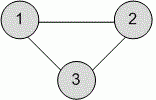Competitions

# From adjacency matrix to the list of edges

The simple not oriented graph is given by the adjacency matrix. Print its representation as a list of edges.

#### Input

The first line contains the number n (1n100) of vertices in a graph. Each of the next n lines contains n elements - the description of adjacency matrix.

#### Output

Print the list of edges, ordered by the first vertex in a pair that describes an edge.Time limit 1 second
Memory limit 128 MiB
Input example #1
3
0 1 1
1 0 1
1 1 0

Output example #1
1 2
1 3
2 3